# Visualize Image Classifications Using Maximal and Minimal Activating Images

This example shows how to use a data set to find out what activates the channels of a deep neural network. This allows you to understand how a neural network works, and also diagnose potential issues with a training data set.

This example covers a number of simple visualization techniques, using a GoogLeNet transfer-learned on a food data set.

By looking at images that maximally or minimally activate the classifier, you learn how to diagnose why a neural network gets classifications wrong using a simple technique based around the class scores for different classes of images.

Load the images as an image datastore. This small data set contains a total of 978 observations with 9 classes of food.

Split this data into a training, validation, and test set to prepare for transfer learning on GoogLeNet. Display a few pictures from the data set.

```rng default dataDir = fullfile(tempdir, "Food Dataset"); url = "https://www.mathworks.com/supportfiles/nnet/data/ExampleFoodImageDataset.zip"; if ~exist(dataDir, "dir") mkdir(dataDir); end downloadExampleFoodImagesData(url,dataDir);```
```Downloading MathWorks Example Food Image dataset... This can take several minutes to download... Download finished... Unzipping file... Unzipping finished... Done. ```
```imds = imageDatastore(dataDir, ... "IncludeSubfolders", true, "LabelSource", "foldernames"); [imdsTrain,imdsValidation, imdsTest] = splitEachLabel(imds, 0.6, 0.2); rnd = randperm(numel(imds.Files), 9); for i = 1:numel(rnd) subplot(3,3,i) imshow(imread(imds.Files{rnd(i)})) label = imds.Labels(rnd(i)); title(label, "Interpreter", "none") end```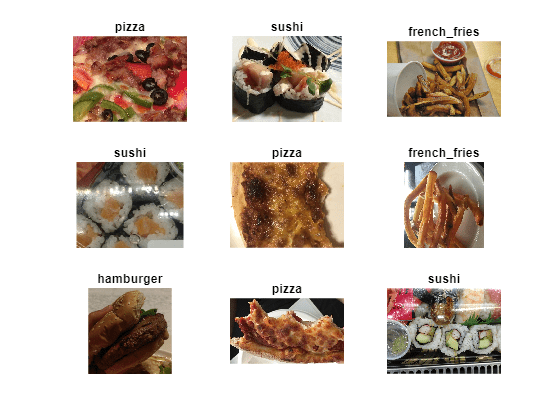Train Network to Classify Food Images

Use the pretrained GoogLeNet network, and train it again to classify the 9 classes of food. If you don't have the Deep Learning Toolbox™ Model for GoogLeNet Network support package installed, then the software provides a download link.

To try a different pretrained network, open this example in MATLAB® and select a different network, such as `squeezenet`, a network that is even faster than `googlenet`. For a list of all available networks, see Load Pretrained Networks.

`net = googlenet;`

The first element of the `Layers` property of the network is the image input layer. This layer requires input images of size 224-by-224-by-3, where 3 is the number of color channels.

`inputSize = net.Layers(1).InputSize;`

The convolutional layers of the network extract image features that the last learnable layer and the final classification layer use to classify the input image. These two layers, `'loss3-classifier'` and `'output'` in GoogLeNet, contain information on how to combine the features that the network extracts into class probabilities, a loss value, and predicted labels. To train again a pretrained network to classify new images, replace these two layers with new layers adapted to the new data set.

Extract the layer graph from the trained network.

`lgraph = layerGraph(net);`

In most networks, the last layer with learnable weights is a fully connected layer. Replace this fully connected layer with a new fully connected layer with the number of outputs equal to the number of classes in the new data set (9, in this example).

```numClasses = numel(categories(imdsTrain.Labels)); newfclayer = fullyConnectedLayer(numClasses,... 'Name', 'new_fc',... 'WeightLearnRateFactor',10,... 'BiasLearnRateFactor',10); lgraph = replaceLayer(lgraph, net.Layers(end-2).Name, newfclayer);```

The classification layer specifies the output classes of the network. Replace the classification layer with a new one without class labels. `trainNetwork` automatically sets the output classes of the layer at training time.

```newclasslayer = classificationLayer('Name', 'new_classoutput'); lgraph = replaceLayer(lgraph, net.Layers(end).Name, newclasslayer);```

Train Network

The network requires input images of size 224-by-224-by-3, but the images in the image datastore have different sizes. Use an augmented image datastore to automatically resize the training images. Specify additional augmentation operations to perform on the training images: randomly flip the training images along the vertical axis and randomly translate them up to 30 pixels and scale them up to 10% horizontally and vertically. Data augmentation helps prevent the network from overfitting and memorizing the exact details of the training images.

```pixelRange = [-30 30]; scaleRange = [0.9 1.1]; imageAugmenter = imageDataAugmenter( ... 'RandXReflection',true, ... 'RandXTranslation',pixelRange, ... 'RandYTranslation',pixelRange, ... 'RandXScale',scaleRange, ... 'RandYScale',scaleRange); augimdsTrain = augmentedImageDatastore(inputSize(1:2),imdsTrain, ... 'DataAugmentation',imageAugmenter);```

To automatically resize the validation images without performing further data augmentation, use an augmented image datastore without specifying any additional preprocessing operations.

`augimdsValidation = augmentedImageDatastore(inputSize(1:2),imdsValidation);`

Specify the training options. Set `InitialLearnRate` to a small value to slow down learning in the transferred layers that are not already frozen. In the previous step, you increased the learning rate factors for the last learnable layer to speed up learning in the new final layers. This combination of learning rate settings results in fast learning in the new layers, slower learning in the middle layers, and no learning in the earlier, frozen layers.

Specify the number of epochs to train for. When performing transfer learning, you do not need to train for as many epochs. An epoch is a full training cycle on the entire training data set. Specify the mini-batch size and validation data. Compute the validation accuracy once per epoch.

```miniBatchSize = 10; valFrequency = floor(numel(augimdsTrain.Files)/miniBatchSize); options = trainingOptions('sgdm', ... 'MiniBatchSize',miniBatchSize, ... 'MaxEpochs',6, ... 'InitialLearnRate',3e-4, ... 'Shuffle','every-epoch', ... 'ValidationData',augimdsValidation, ... 'ValidationFrequency',valFrequency, ... 'Verbose',false, ... 'Plots','training-progress');```

Train the network using the training data. By default, `trainNetwork` uses a GPU if one is available. This requires the Parallel Computing Toolbox™ and a CUDA® enabled GPU with compute capability 3.0 or higher. Otherwise, `trainNetwork` uses a CPU. You can also specify the execution environment by using the `'ExecutionEnvironment'` name-value pair argument of `trainingOptions`. Because this data set is small, the training is fast. If you run this example and train the network yourself, you will get different results and misclassifications caused by the randomness involved in the training process.

`net = trainNetwork(augimdsTrain,lgraph,options);`Classify Test Images

Classify the test images using the fine-tuned network, and calculate the classification accuracy.

```augimdsTest = augmentedImageDatastore(inputSize(1:2), imdsTest); [predictedClasses, predictedScores] = classify(net, augimdsTest); accuracy = mean(predictedClasses == imdsTest.Labels)```
```accuracy = 0.8622 ```

### Confusion Matrix for the Test Set

Plot a confusion matrix of the test set predictions. This highlights which particular classes cause most problems for the network.

```figure; confusionchart(imdsTest.Labels, predictedClasses, 'Normalization', "row-normalized");```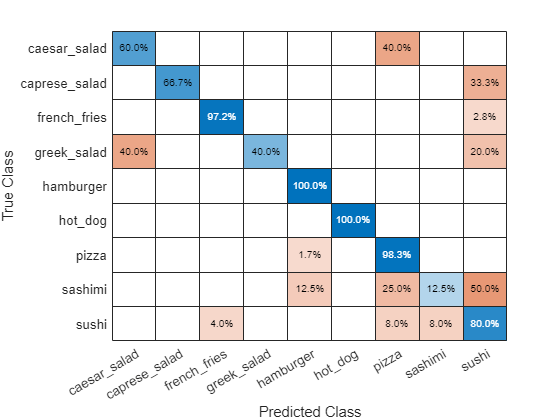The confusion matrix shows that, most of the time, the network is incorrectly classifying some classes, such as greek salad, sashimi, and sushi. To better understand why this is happening, run some tests on these 3 classes, while keeping in mind that the data set used in this example underrepresents them.

```figure(); histogram(imdsValidation.Labels); ax = gca(); ax.XAxis.TickLabelInterpreter = "none";```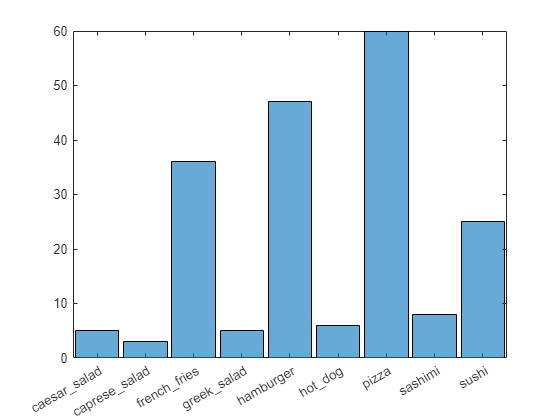Sushis Most Like Sushi

First, find which images of sushi most strongly activate the network for the sushi class. This answers the question "Which images does the network think are most sushi-like?".

To do this, plot the maximally-activating images, or the input images that strongly activate the fully-connected layer's "sushi" neuron. This figure shows the top 4 images, in a descending class score.

```chosenClass = "sushi"; classIdx = find(net.Layers(end).Classes == chosenClass); numImgsToShow = 4; [sortedScores, imgIdx] = findMaxActivatingImages(imdsTest, chosenClass, predictedScores, numImgsToShow); figure plotImages(imdsTest, imgIdx, sortedScores, predictedClasses, numImgsToShow)```Visualize Cues for the Sushi Class

Is the network looking at the right thing for sushi? The maximally-activating images of the sushi class for the network all look similar to each other - a lot of round shapes clustered closely together.

The network is doing well at classifying those kinds of sushis. However, to verify that this is true and to better understand why the network makes its decisions, use a visualization technique like Grad-CAM. For more information on using Grad-CAM, see Grad-CAM Reveals the Why Behind Deep Learning Decisions.

To use Grad-CAM, create a `dlnetwork` from the GoogLeNet network. Specify the name of the softmax layer, `'prob'`. Specify the name of the final convolutional layer, `'inception_5b-output'`.

```lgraph = layerGraph(net); lgraph = removeLayers(lgraph, lgraph.Layers(end).Name); dlnet = dlnetwork(lgraph); softmaxName = 'prob'; convLayerName = 'inception_5b-output';```

Read the first resized image from the augmented image datastore , then plot the Grad-CAM visualization.

```imageNumber = 1; observation = augimdsTest.readByIndex(imgIdx(imageNumber)); img = observation.input{1}; label = predictedClasses(imgIdx(imageNumber)); score = sortedScores(imageNumber); gradcamMap = computeGradCAM(dlnet, img, softmaxName, convLayerName, label); figure alpha = 0.5; plotGradCAM(img, gradcamMap, alpha); sgtitle(string(label)+" (score: "+ max(score)+")")```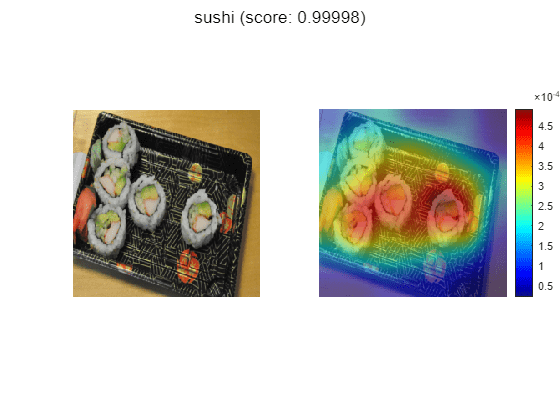The plot confirms that the network is correctly focusing on sushi instead of the plate or the table. The network classifies this image as sushi because it sees a group of sushis. However, is it able to classify one sushi on its own?

The second image has a cluster of sushi on the left and a lone sushi on the right. To see what the network focuses on, read the second image and plot the Grad-CAM.

```imageNumber = 2; observation = augimdsTest.readByIndex(imgIdx(imageNumber)); img = observation.input{1}; label = predictedClasses(imgIdx(imageNumber)); score = sortedScores(imageNumber); gradcamMap = computeGradCAM(dlnet, img, softmaxName, convLayerName, label); alpha = 0.5; figure plotGradCAM(img, gradcamMap, alpha); sgtitle(string(label)+" (score: "+ max(score)+")")```The network associates the sushi class with multiple sushis stacked together. Test this by looking at a picture of just one sushi.

```img = imread(strcat(tempdir,"Food Dataset/sushi/sushi_18.jpg")); img = imresize(img, net.Layers(1).InputSize(1:2), "Method", "bilinear", "AntiAliasing", true); [label,score] = classify(net, img); gradcamMap = computeGradCAM(dlnet, img, softmaxName, convLayerName, label); figure alpha = 0.5; plotGradCAM(img, gradcamMap, alpha); sgtitle(string(label)+" (score: "+ max(score)+")")```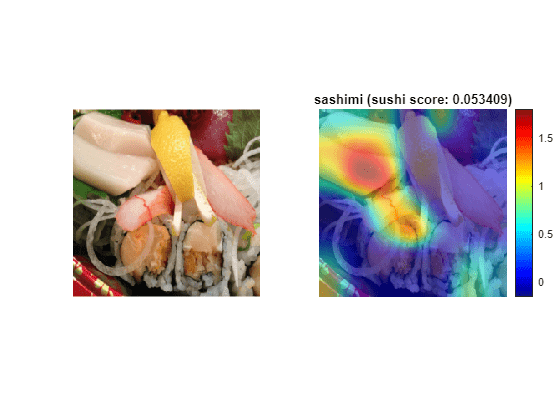The network is able to classify this lone sushi correctly. However, the GradCAM shows that the network focuses more on the cluster of cucumber in the sushi rather than the whole piece together.

Run the Grad-CAM visualization technique on a lone sushi that does not contain any stacked small pieces of ingredients.

```img = imread("crop__sushi34-copy.jpg"); img = imresize(img, net.Layers(1).InputSize(1:2), "Method", "bilinear", "AntiAliasing", true); [label,score] = classify(net, img); gradcamMap = computeGradCAM(dlnet, img, softmaxName, convLayerName, label); figure alpha = 0.5; plotGradCAM(img, gradcamMap, alpha); title(string(label)+" (score: "+ max(score)+")")```In this case, the visualization technique highlights why the network performs poorly. It incorrectly classifies the image of the sushi as a hamburger.

To solve this issue, you have to feed the network with more images of lone sushi during the training process.

Sushis Least Like Sushis

Now find which images of sushi activate the network for the sushi class the least. This answers the question "Which images does the network think are less sushi-like?".

This is useful because it finds the images on which the network performs badly, and it provides some insight into its decision.

```chosenClass = "sushi"; numImgsToShow = 9; [sortedScores, imgIdx] = findMinActivatingImages(imdsTest, chosenClass, predictedScores, numImgsToShow); figure plotImages(imdsTest, imgIdx, sortedScores, predictedClasses, numImgsToShow)```Investigate Sushi Misclassified as Sashimi

Why is the network classifying sushi as sashimi? The network classifies 3 out of the 9 images as sashimi, respectively images 2, 4, and 9. Two of them, images 4 and 9, actually contain sashimi, which means the network isn't actually misclassifying them. These images are mislabeled.

To see what the network is focusing on, run the Grad-CAM technique on one of these images .

```imageNumber = 2; observation = augimdsTest.readByIndex(imgIdx(imageNumber)); img = observation.input{1}; label = predictedClasses(imgIdx(imageNumber)); score = sortedScores(imageNumber); gradcamMap = computeGradCAM(dlnet, img, softmaxName, convLayerName, label); figure alpha = 0.5; plotGradCAM(img, gradcamMap, alpha); title(string(label)+" (sushi score: "+ max(score)+")")```As expected, the network focuses on the sashimi instead of the sushi.

Investigate Sushi Misclassified as Pizza

Why is the network classifying sushi as pizza? The network classifies 4 out of 9 images as pizza instead of sushi. These images have many colorful toppings.

To see which part of the image the network is looking at, run the Grad-CAM technique on one of these images.

```imageNumber = 5; observation = augimdsTest.readByIndex(imgIdx(imageNumber)); img = observation.input{1}; label = predictedClasses(imgIdx(imageNumber)); score = sortedScores(imageNumber); gradcamMap = computeGradCAM(dlnet, img, softmaxName, convLayerName, label); figure alpha = 0.5; plotGradCAM(img, gradcamMap, alpha); title(string(label)+" (sushi score: "+ max(score)+")")```The network strongly focuses on the toppings.

To help the network distinguish pizza from sushi with toppings, add more training images of sushi with toppings.

Investigate Sushi Misclassified as French Fries

Why is the network classifying sushi as french fries? The network classifies the 7th image as french fries instead of sushi. This specific sushi is yellow and the network might associate this color with french fries.

```imageNumber = 7; observation = augimdsTest.readByIndex(imgIdx(imageNumber)); img = observation.input{1}; label = predictedClasses(imgIdx(imageNumber)); score = sortedScores(imageNumber); gradcamMap = computeGradCAM(dlnet, img, softmaxName, convLayerName, label); figure alpha = 0.5; plotGradCAM(img, gradcamMap, alpha); title(string(label)+" (sushi score: "+ max(score)+")", "Interpreter", "none")```The networks focuses on the yellow sushi classifying it as french fries.

To help the network in this specific case, train it with more images of yellow foods that are not french fries.

Most and Least Like a Sashimi

The food data set comprises only 8 observations of sashimi.

Similar to the sushi class, display the sashimi images in order of most like sashimi to least like sashimi.

```chosenClass = "sashimi"; numImgsToShow = 8; [sortedScores, imgIdx] = findMaxActivatingImages(imdsTest, chosenClass, predictedScores, numImgsToShow); figure plotImages(imdsTest, imgIdx, sortedScores, predictedClasses, numImgsToShow)```This figure shows that the network is not confident about sashimi accompanied by certain other food, such as sashimi with greenery. However this also shows that there are some flaws in the data where pictures of sushi are mislabeled as sashimi as ground truth such as image number 5.

Visualize Cues for the Sashimi Class

Is the network looking at the right thing for sashimi? Consider only the images that the network correctly classifies as sashimi, and check what it focuses on during the classification process.

```imageNumber = 1; observation = augimdsTest.readByIndex(imgIdx(imageNumber)); img = observation.input{1}; label = predictedClasses(imgIdx(imageNumber)); score = sortedScores(imageNumber); gradcamMap = computeGradCAM(dlnet, img, softmaxName, convLayerName, label); figure alpha = 0.5; plotGradCAM(img, gradcamMap, alpha); title(string(label)+" (score: "+ max(score)+")")```This image contains both sashimi and sushi. The network correctly focuses on the sashimi and ignores the sushi. However, the data set contains other images that also have a mix of sushi and sashimi but they are labeled as sushi-only instead. This makes it hard for the network to learn how to properly classify these images, as shown in the "sushi least like sushi" case. This also explains why the prediction score isn't very high in this particular case.

Investigate Sashimi Misclassified as Hamburger

Why is the network classifying sashimi as hamburger? The network classifies 3 out of 8 images of sashimi as hamburger. The data set contains many more observations of hamburger that might bias the network.

```imageNumber = 4; observation = augimdsTest.readByIndex(imgIdx(imageNumber)); img = observation.input{1}; label = predictedClasses(imgIdx(imageNumber)); score = sortedScores(imageNumber); gradcamMap = computeGradCAM(dlnet, img, softmaxName, convLayerName, label); figure alpha = 0.5; plotGradCAM(img, gradcamMap, alpha); title(string(label)+" (sashimi score: "+ max(score)+")")```Here, the seaweed-wrapped food confuses the network, as it resembles the bun-meat-bun characteristics of a humburger. This again is a flaw in the sashimi data which is not varied enough.

Investigate Sashimi Misclassified as Greek Salad

Why is the network classifying sashimi as greek salad? The network classifies the 6th image as greek instead of sashimi.

```imageNumber = 6; observation = augimdsTest.readByIndex(imgIdx(imageNumber)); img = observation.input{1}; label = predictedClasses(imgIdx(imageNumber)); score = sortedScores(imageNumber); gradcamMap = computeGradCAM(dlnet, img, softmaxName, convLayerName, label); figure alpha = 0.5; plotGradCAM(img, gradcamMap, alpha); title(string(label)+" (sashimi score: "+ max(score)+")", "Interpreter", "none")```Here, the greenery misleads the network into thinking that the images depicts a plate of sashimi. To help the network recognize the difference between these two classes, add a more varied data set of sashimi accompanied by leaves and vegetables.

Most and Least Like a Greek Salad

The food data set only comprises 5 observations of greek salad.

Similar to the above cases, display the greek salad images in order of most likely greek salad to least likely greek salad.

```chosenClass = "greek_salad"; numImgsToShow = 5; [sortedScores, imgIdx] = findMaxActivatingImages(imdsTest, chosenClass, predictedScores, numImgsToShow); figure plotImages(imdsTest, imgIdx, sortedScores, predictedClasses, numImgsToShow)```This figure shows that the network is not confident about greek salad accompanied by certain other food, such as meat.

Investigate Cues for the Greek Salad Class

Is the network looking at the right thing for greek salad? Consider only the images that the network correctly classifies as greek salad, and check what it focuses on during the classification process.

```imageNumber = 1; observation = augimdsTest.readByIndex(imgIdx(imageNumber)); img = observation.input{1}; label = predictedClasses(imgIdx(imageNumber)); score = sortedScores(imageNumber); gradcamMap = computeGradCAM(dlnet, img, softmaxName, convLayerName, label); figure alpha = 0.5; plotGradCAM(img, gradcamMap, alpha); title(string(label)+" (score: "+ max(score)+")", "Interpreter", "none")```The network ignores the actual greek salad and rather looks at things in the background instead. This is an indication that the network is classifying this image correctly but for the wrong reasons.

```imageNumber = 2; observation = augimdsTest.readByIndex(imgIdx(imageNumber)); img = observation.input{1}; label = predictedClasses(imgIdx(imageNumber)); score = sortedScores(imageNumber); gradcamMap = computeGradCAM(dlnet, img, softmaxName, convLayerName, label); figure alpha = 0.5; plotGradCAM(img, gradcamMap, alpha); title(string(label)+" (score: "+ max(score)+")", "Interpreter", "none")```Even though greek salad usually does not have eggs, the network focuses on the tomato and olives which indicates it is looking at the right things for a greek salad.

Investigate Greek Salad Misclassified as Hamburger

Why is the network classifying greek salad as hamburger? The network classifies the fourth image as a hamburger. Is it caused by the chunks of meat in the salad?

```imageNumber = 4; observation = augimdsTest.readByIndex(imgIdx(imageNumber)); img = observation.input{1}; label = predictedClasses(imgIdx(imageNumber)); score = sortedScores(imageNumber); gradcamMap = computeGradCAM(dlnet, img, softmaxName, convLayerName, label); figure alpha = 0.5; plotGradCAM(img, gradcamMap, alpha); title(string(label)+" (greek_salad score: "+ max(score)+")", "Interpreter", "none")```The network focuses on the regions with meat.

Conclusion

Investigating the datapoints that give rise to large or small class scores, and datapoints that the network classifies confidently but incorrectly, is a simple technique which can provide useful insight into how a trained network is functioning. In the case of the food data set, this example highlighted that:

• The test data contains a number of images with incorrect true labels, such as the "sashimi" which is actually "sushi" instead.

• The network considers a "sushi" to be "multiple, clustered, round-shaped things". However, it must be able to distinguish a lone sushi as well.

• Any sushi or sashimi with toppings or unusual colors confuses the network. To resolve this problem, the data must have a wider variety of sushi and sashimi.

• Any sushi or sashimi accompanied by salad-like ingredients is likely to be confused with salad classes. Therefore, you must train the network with more images of sushi and sashimi with some salad on the side.

• To learn what makes a greek salad stands out, the network needs more observations of greek salads.

### Helper functions

```function downloadExampleFoodImagesData(url, dataDir) % Download the Example Food Image data set, containing 978 images of % different types of food split into 9 classes. % Copyright 2019 The MathWorks, Inc. fileName = "ExampleFoodImageDataset.zip"; fileFullPath = fullfile(dataDir, fileName); % Download the .zip file into a temporary directory. if ~exist(fileFullPath, "file") fprintf("Downloading MathWorks Example Food Image dataset...\n"); fprintf("This can take several minutes to download...\n"); websave(fileFullPath, url); fprintf("Download finished...\n"); else fprintf("Skipping download, file already exists...\n"); end % Unzip the file. % % Check if the file has already been unzipped by checking for the presence % of one of the class directories. exampleFolderFullPath = fullfile(dataDir, "pizza"); if ~exist(exampleFolderFullPath, "dir") fprintf("Unzipping file...\n"); unzip(fileFullPath, dataDir); fprintf("Unzipping finished...\n"); else fprintf("Skipping unzipping, file already unzipped...\n"); end fprintf("Done.\n"); end function [sortedScores, imgIdx] = findMaxActivatingImages(imds, className, predictedScores, numImgsToShow) % Find the predicted scores of the chosen class on all the images of the chosen class % (e.g. predicted scores for sushi on all the images of sushi) [scoresForChosenClass, imgsOfClassIdxs] = findScoresForChosenClass(imds, className, predictedScores); % Sort the scores in descending order [sortedScores, idx] = sort(scoresForChosenClass, 'descend'); % Return the indices of only the first few imgIdx = imgsOfClassIdxs(idx(1:numImgsToShow)); end function [sortedScores, imgIdx] = findMinActivatingImages(imds, className, predictedScores, numImgsToShow) % Find the predicted scores of the chosen class on all the images of the chosen class % (e.g. predicted scores for sushi on all the images of sushi) [scoresForChosenClass, imgsOfClassIdxs] = findScoresForChosenClass(imds, className, predictedScores); % Sort the scores in ascending order [sortedScores, idx] = sort(scoresForChosenClass, 'ascend'); % Return the indices of only the first few imgIdx = imgsOfClassIdxs(idx(1:numImgsToShow)); end function [scoresForChosenClass, imgsOfClassIdxs] = findScoresForChosenClass(imds, className, predictedScores) % Find the index of className (e.g. "sushi" is the 9th class) uniqueClasses = unique(imds.Labels); chosenClassIdx = string(uniqueClasses) == className; % Find the indices in imageDatastore that are images of label "className" % (e.g. find all images of class sushi) imgsOfClassIdxs = find(imds.Labels == className); % Find the predicted scores of the chosen class on all the images of the % chosen class % (e.g. predicted scores for sushi on all the images of sushi) scoresForChosenClass = predictedScores(imgsOfClassIdxs,chosenClassIdx); end function plotImages(imds, imgIdx, sortedScores, predictedClasses,numImgsToShow) for i=1:numImgsToShow score = sortedScores(i); sortedImgIdx = imgIdx(i); predClass = predictedClasses(sortedImgIdx); correctClass = imds.Labels(sortedImgIdx); imgPath = imds.Files{sortedImgIdx}; if predClass == correctClass color = "\color{green}"; else color = "\color{red}"; end subplot(3,ceil(numImgsToShow./3),i) imshow(imread(imgPath)); title("Predicted: " + color + string(predClass) + "\newline\color{black}Score: " + num2str(score) + "\newlineGround truth: " + string(correctClass)); end end function [convMap,dScoresdMap] = gradcam(dlnet, dlImg, softmaxName, convLayerName, classfn) % Computes the Grad-CAM map for a dlnetwork, taking the derivative of the softmax layer score % for a given class with respect to a convolutional feature map. [scores,convMap] = predict(dlnet, dlImg, 'Outputs', {softmaxName, convLayerName}); classScore = scores(classfn); dScoresdMap = dlgradient(classScore,convMap); end function gradcamMap = computeGradCAM(dlnet, img, softmaxName, convLayerName, label) % For automatic differentiation, the input image img must be a dlarray. dlImg = dlarray(single(img),'SSC'); % Compute the gradCAM map by passing the dlarray image [convMap, dScoresdMap] = dlfeval(@gradcam, dlnet, dlImg, softmaxName, convLayerName, label); % Resize the gradient map to the net image size, and scale the scores to the appropriate levels for display. gradcamMap = sum(convMap .* sum(dScoresdMap, [1 2]), 3); gradcamMap = extractdata(gradcamMap); gradcamMap = rescale(gradcamMap); gradcamMap = imresize(gradcamMap, dlnet.Layers(1).InputSize(1:2), 'Method', 'bicubic'); end function plotGradCAM(img, gradcamMap, alpha) subplot(1,2,1) imshow(img); h= subplot(1,2,2); imshow(img) hold on; imagesc(gradcamMap,'AlphaData', alpha); originalSize2 = get(h, 'Position'); colormap jet colorbar set(h, 'Position', originalSize2); hold off; end ```